Know the function of a fuse box and the appearance of a tripped circuit breaker – Marilyn Vos Savant

## Oscillators

What determines how fast your clock is ticking ? Waveform refers to the shape and form of a signal such as a wave moving in a physical medium. Oscillators help you to generate a repetitive waveform of desired frequency , amplitude and shape. The waveform can be square, sinusoidal or triangular waveform, as shown :

• Sine• Square• Triangle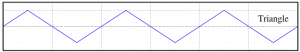• Sawtooth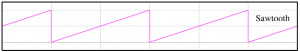Oscillators or waveform generators are used in digital multimeters, oscilloscopes, computers and much much more. I have an FG-100 DDS function generator which is one of the cheapest function generators to generate sine, square, sawtooth and other waveforms and I’m gonna show you how we generate waveforms in the following video :

## Relaxation Oscillators

It is the easiest type of oscillator to design which charges a capacitor through a resistor and then rapidly discharges it when the capacitor voltage reaches a certain threshold voltage ( cycle repeats continuously ). < An explanation and derivation will be provided once we start OpAmps >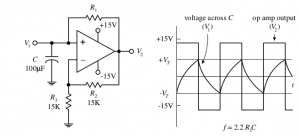## 555 Timer IC

The 555 timer IC is a precision timer that can act either as a timer or an oscillator and gets its name from the three 5k ohm resistors in the block which act as a three-step voltage divider between Vcc ( supply voltage ) and ground. The two comparators output either a high / low voltage based on the analog voltages being being compared at their inputs. if one of the comparator’s positive input is more positive than its negative input, its output logic level goes high; if the positive input voltage is less than the negative input voltage, the output logic level goes low.  The output of the comparators are sent to the input of an SR flip-fop. The flip-flop looks at R and S inputs and produces either a high / low based on the voltage states of the inputs.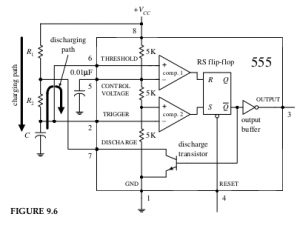Pin 1 : IC GND

Pin 2 (trigger) : Input to comparator 2 to set the flip-flop. When the voltage at pin 2 crosses from above to below Vcc/3 ,the comparator switches to high, setting the flip-flop.

Pin 3 (output) : Output of 555 driven by an inverting buffer capable of sinking or sourcing around 200mA.

Pin 4 (reset) : Active-low reset, which forces Q’ (in SR flip-flop) hugh and output low.

Pin 5 (control) : Used to override the 2/3Vcc level, if needed, but is usually grounded via a bypass capacitor.

Pin 6 (threshold) : Input to upper comparator, which is used to set the flip-flop. When the voltage at pin 6 crosses from below to above 2/3Vcc, the comparator switches to high, resetting the flip-flop.

Pin 7 (discharge) : Connected to the open collector of npn traansistor and is used to short pin 7 to ground when Q’ is high (pin3 low).

Pin 8 (Vcc) : Typically between 4.5-16V for general-purpose TTL 555s.

In the astable configuration, when power is first applied to the system, the capacitor is uncharged. This means that 0 V is placed on pin 2, forcing comparator 2 high. This in turn sets the flip-flop so that Q is high and the 555’s output is low (a result of the inverting buffer). With Q high, the discharge transistor is turned on, which allows the capacitor to charge toward Vcc through R1 and R2 . When the capacitor voltage exceeds 1/3Vcc , comparator 2 goes low, which has no effect on the SR flip-flop. However, when the capacitor voltage exceeds 2/3Vcc , comparator 1 goes high, resetting the flip-flop and forcing Q high and the output low. At this point, the discharge transistor turns on and shorts pin 7 to ground, discharging the capacitor through R2 . When the capacitor’s voltage drops below 1/3Vcc , comparator 2’s output jumps back to a high level, setting the flip-flop and making Q low and the output high. With Q low, the transistor turns on, allowing the capacitor to start charging again. The cycle repeats over and over again. The net result is a squarewave output pattern whose voltage level is approximately Vcc − 1.5V and whose on/off periods are determined by the C, R1 and R2. The circuit given below oscillates between 3V and 6V.

## Parts Required

1.  BC547 Transistor
2.  Jumper Wires
3.  470 Ohm Resistor
4.  100uF Capacitor
5.  10k Ohm Potentiometer
6.  LED
7.  9V Battery or a Power Supply
9.  555 IC

## Circuit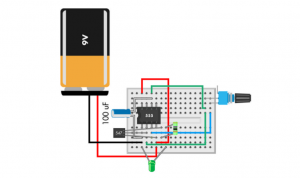## What do you see ?